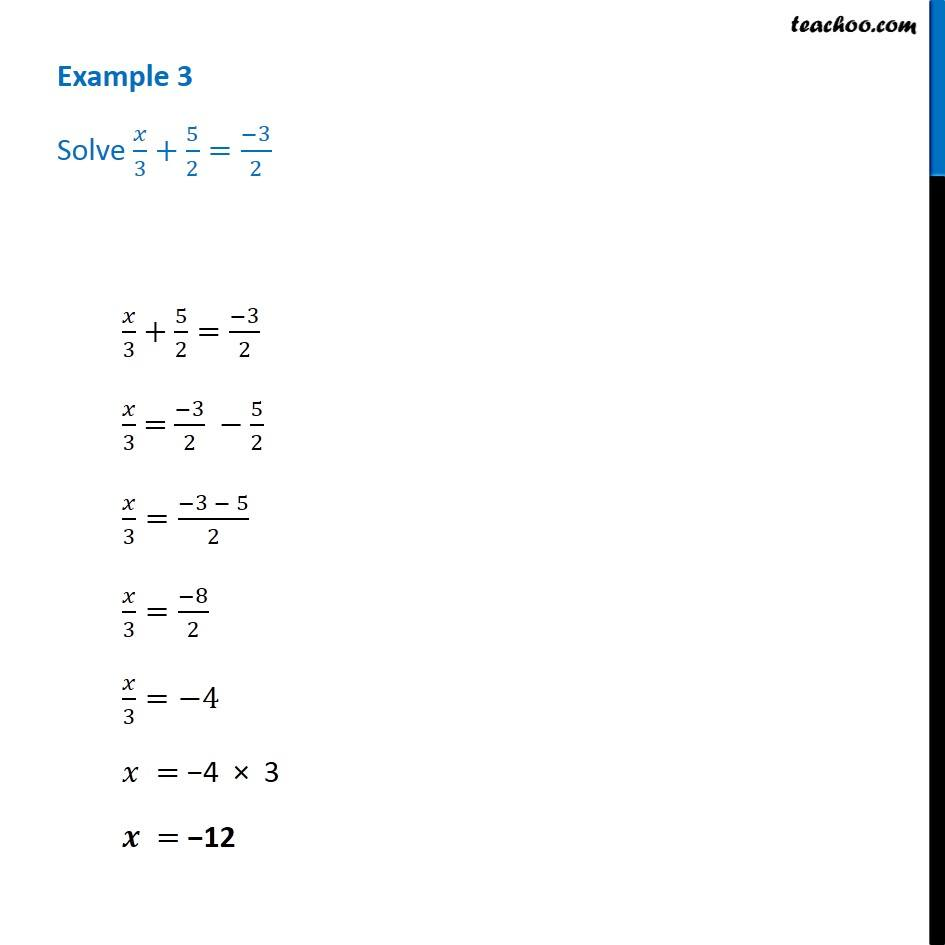Examples

Chapter 2 Class 8 Linear Equations in One Variable
Serial order wiseLearn in your speed, with individual attention - Teachoo Maths 1-on-1 Class

### Transcript

Question 3 - Chapter 2 Class 8 - Linear Equations in one variable - NCERT Book Solve x/3+5/2 = (-3)/2 x/3 + 5/2 = (-3)/2 x/3 = (-3)/2 5/2 x/3=(-3 - 5)/2 x/3=( 8)/2 x/3= 4 x = 4 × 3 x = 12